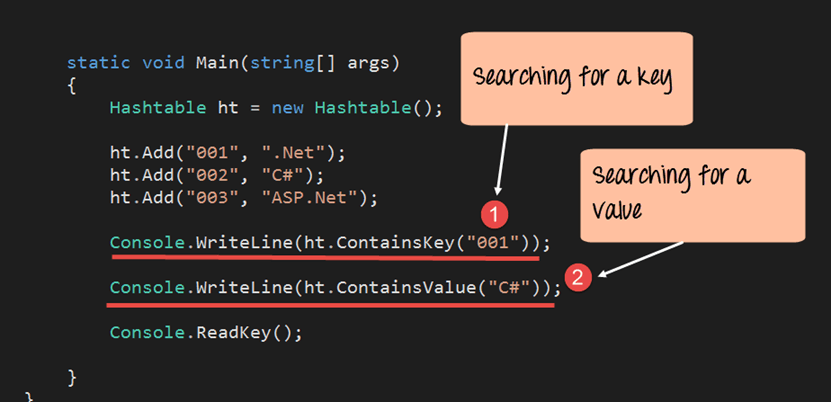## What is Hashtable in C#?

A hash table is a special collection that is used to store key-value items. So instead of storing just one value like the stack, array list and queue, the hash table stores 2 values. These 2 values form an element of the hash table.

Below are some example of how values of a hash table might look like.

```{ "001" , ".Net" }
{ "002" , ".C#" }
{ "003" , "ASP.Net" }```

Above we have 3 key value pairs. The keys of each element are 001, 002 and 003 respectively. The values of each key value pair are ".Net", "C#" and "ASP.Net" respectively.

Let's look at the operations available for the Hashtable collection in more detail.

### Declaration of the Hashtable

The declaration of a Hashtable is shown below. A Hashtable is created with the help of the Hashtable Datatype. The "new" keyword is used to create an object of a Hashtable. The object is then assigned to the variable ht.

`Hashtable ht = new Hashtable()`

### Adding elements to the Hashtable

The Add method is used to add an element on to the queue. The general syntax of the statement is given below

`HashTable.add("key","value")`

### Example 1:

Remember that each element of the hash table comprises of 2 values, one is the key, and the other is the value.

Now, let's see this working at a code level. All of the below-mentioned code will be written to our Console application.

The code will be written to our Program.cs file. In the below program, we will write the code to see how we can use the above-mentioned methods.

For now in our example, we will just look at how we can create a hashtable , add elements to the hashtable and display them accordingly.

```using System;
using System.Collections;
using System.Collections.Generic;
using System.Linq;
using System.Text;

namespace DemoApplication
{
class Program
{
static void Main(string[] args)
{
Hashtable ht = new Hashtable();

ICollection keys = ht.Keys;

foreach (String k in keys)
{
Console.WriteLine(ht[k]);
}
}
}
} ```

Code Explanation:-

1. First, we declare the hashtable variable using the Hashtable data type by using keyword "New." The name of the variable defines is 'ht'.
2. We then add elements to the hash table using the Add method. Remember that we need to add both a key and value element when adding something to the hashtable.
3. There is no direct way to display the elements of a hash table.
• In order to display the hashtable , we first need to get the list of keys (001, 002 and 003) from the hash table.
• This is done via the ICollection interface. This is a special data type which can be used to store the keys of a hashtable collections. We then assign the keys of the hashtable collection to the variable 'keys'.
1. Next for each key value, we get the associated value in the hashtable by using the statement ht[k].

If the above code is entered properly and the program is run the following output will be displayed.

Output:

Let's look at some more methods available for hash tables.

### ContainsKey

This method is used to see if a key is present in the Hashtable. Below is the general syntax of this statement. The statement will return true if the key exists, else it will return the value false.

`Hashtable.Containskey(key)`

### ContainsValue

This method is used to see if a Value is present in the Hashtable. Below is the general syntax of this statement. The statement will return true if the Value exists, else it will return the value false.

`Hashtable.ContainsValue(value)`

### Example 2:

Let's change the code in our Console application to showcase how we can use the "Containskey" and "ContainsValue" method.```using System;
using System.Collections;
using System.Collections.Generic;
using System.Linq;
using System.Text;

namespace DemoApplication
{
class Program
{
static void Main(string[] args)
{
Hashtable ht = new Hashtable();

Console.WriteLine(ht.ContainsKey("001"));
Console.WriteLine(ht.ContainsValue("C#"));
}
}
}```

Code Explanation:-

1. First, we use the ContainsKey method to see if the key is present in the hashtable. This method will return true if the key is present in the hashtable. This method should return true since the key does exist in the hashtable.
2. We then use the ContainsValue method to see if the value is present in the hashtable. This method will return 'true' since the Value does exist in the hashtable.

If the above code is entered properly and the program is run the following output will be displayed.

Output:

From the output, you can clearly see that both the key and value being searched are present in the hash table.

Summary

• A Hashtable is used to store elements which comprises of key values pairs. To access the value of an element , you need to know the key of the element.

YOU MIGHT LIKE: